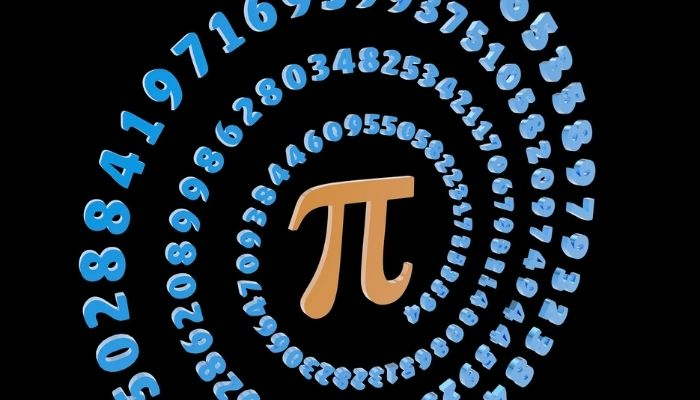# Explain the Concept of Area and Circumference of a Circle

In the planet we live it is spherical, moving around a circular orbit around a sun which is spherical again accompanied by other planets which too move along in circular orbits and are all spherical and to top it all, the earth also has a satellite which again is spherical and moving in a circular orbit around the earth. We must have heard terms like the circumference of a circle commonly in geometry which came into existence because of this shape.

So the above summary of the celestial bodies was only to bring about the point of the circle and its heavenly importance ( no pun intended). For all the shapes we see and can create, it is the shape of the circle chosen by nature for any celestial body almost, and thus it is natural for human beings to study well about a circle and its properties and to calculate the measure of a circle with the help of the area of a circle formula.

A circle is often defined as a locus that gets created when a point moves around a fixed point and keeps a fixed distance; the path thus carved is a circle, and the fixed point is called the center of the circle, and the fixed distance is the radius of the circle. For a circle, all the points along its periphery are at a fixed distance from the center of the circle. This is called the radius of the circle and is pretty much the only thing one needs to know about a circle to draw it. As against many kinds of triangles and polygons available, there is only one type of circle, and the only difference between circles is the length of the radius.

A circle is a two-dimensional figure, and it has a curved line that makes up a closed space. The amount of space thus enclosed by this curved line is called the area of the circle, and the length of the curved line is called the circumference of the circle. As circles have been fascinating to the mankind since the beginning, remember the earliest invention of the wheel was circular too. Thus it was noticed by many that the length of the periphery of the circle when measured manually always had a constant ratio with twice its radius. It is important to note here that twice the radius is also called the diameter of the circle, which is essentially the line that divides the circle into two equal halves. The diameter connects two points in a circle and passes through the center. So, it was noticed long back that length of the periphery of the circle, when divided by the diameter, always gives a fixed value, and this fixed value was later known as the famous constant ‘pi,’ the symbol of which isThe circumference of the circle is the same as the length of the periphery, and its length can be calculated using the constant pi as pi * diameter or  2 * pi * radius. To calculate the area of a circle which implies the amount of surface enclosed by the periphery. One can also imagine the area of a circle as the summation of the periphery of many concentric circles from radius r to radius zero. Thus, if one integrates the circumference from radius r to zero, one would get the area of the circle. The formula to find the area of a circle isr2.

If there is a circular stage, then to know how many tiles would be required for it, we would need to know the area of the circle and divide it by the area of individual tiles, this would help us know how many tiles would be required for the circular stage. Knowing how to calculate the area of a circle helps us in everyday life, and the easy way to calculate the area using the formula is very convenient. Visit the Cuemath website for more examples like this in circle worksheets.